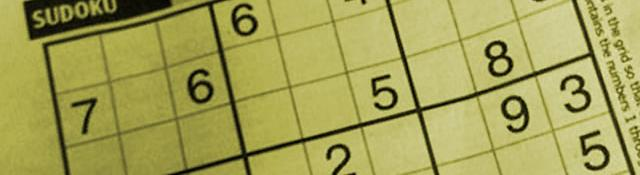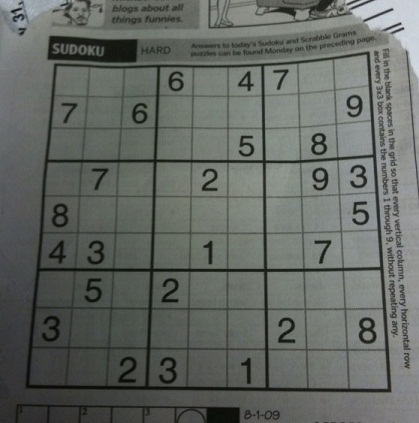## SuDoKu Grabber in OpenCV

I decided to write a quick and fun project. The idea is simple - capture an image, identify the sudoku grid + digits and then solve the puzzle!

## The Plot

Series: SuDoKu Grabber in OpenCV:

Here's an interesting project that I'll be taking your over the next few days. We'll create an app that can recognize a SuDoKu puzzle from a picture taken by a camera (maybe on a phone). We'll be going over a lot of stuff: geometric transformations, character recognition, logic, etc. This post is an overview of how things will work for the SuDoKu grabber!

## Recognizing SuDoKu puzzles

For identifying SuDoKu puzzles, we'll make use of the simpler tools image processing provides. Character recognition, detecting lines, fixing skewed pictures, etc.

Here's a little walkthough of how we'll be implementing things.

Suppose we start off with this particular image:The original puzzle, as seen by the camera

The very first thing we need to do is identify the puzzle. Here are some of the challenges when doing this:

• The inner grid lines are very fine and will probably be tough to recognize.
• The lines are not perfect. We'll have to take care of that.
• The black grid lines have colors same as a lot of other elements in the picture.

To overcome all these, we'll make one assumption: the puzzle is the biggest element in the image. And it's a safe assumption to make. You probably aren't looking to grab a sudoku puzzle if you're looking at an entire newspaper page.

Another assumption I'm using for this series is that there is a thick black border outside the puzzle. In the above picture, the 3x3 boxes too have thick grid lines. But those aren't needed. Only the outermost edges of the sudoku puzzle should have thick dark borders.

### Step 1: Segmenting the SuDoKu puzzle

The first thing we do is segment the puzzle image with thresholding. After this operation, we get the dark regions of the puzzle:

Of course, there is some preprocessing involved. Things like smoothing out noise, some morphological operations, etc. We'll see the details when we start implementing this.

### Step 2: Detecting the puzzle blob

We can't detect lines in the image just yet. There are just way too many outliers. There are the numbers, the writing on the top and right edge of the puzzle. There are lines outside the puzzle too.

So we'll use the assumption that the puzzle is the biggest thing in the image. We check the size of all blobs on the image. Only the puzzle's grid lines are big enough to cover the largest area on the image.

So, on selecting the blob that covers the biggest region, we get this:

This looks great! Next we detect lines in this noise-free image!

### Step 3: Locating the puzzle

We can use the Hough transform to get lines in this image. It returns lines in mathematical terms. So after this step, we'll know exactly where a lines lies... and not just the pixels where it lies.

We don't need all these lines. So we just take the lines nearest to the edges. And because they are mathematical lines, we can solve for their intersection. That could give us the four corners of the puzzle. Then, we know exactly where the puzzle is.

For our example, it looks like this:

The corners are not accurate. But pretty close. They should be good enough.

### Step 4: Fixing the image and accessing each cell

We have four corners. We can remap this into a new image - where each corner corresponds to a corner on the image:

After remapping, we can divide the puzzle into a 9x9 grid. Each cell in the grid will correspond (approximately) to a cell on the puzzle. The correspondence might not be perfect, but it should be good enough.

### Step 5: Identify the numbers

Now for the final part: character recognition. This is simple: we just iterate through each cell and check if it contains some number. If it does, we store it in the internal data structure that holds the sudoku.

## More in the series

This tutorial is part of a series called SuDoKu Grabber in OpenCV:

Utkarsh Sinha created AI Shack in 2010 and has since been working on computer vision and related fields. He is currently at Microsoft working on computer vision.